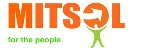﻿
Monday, 24 December 2012 13:56

## validate credit card in c#

Written by
Rate this item

Nowadays credit cards are used in online transactions.almost all
kinds of online transactions involve credit cards & debit cards.

while working with these types of online financial applications,
we sometimes need to validate the credit card or debit card.you
can validate the following cards using c# programming language in

the following c# function will return true if the card is valid,
else it will return false.

you have to pass the card type and number in the following function
-
public bool ValidateCreditCard(string cardType, string cardNumber)
{
byte[] number = new byte;
int length = 0;
for (int j = 0; j < card_number.Length; j++){
if (char.IsDigit(cardNumber, j)){
if (length == 16) return false;
number[length++] = byte.Parse(cardNumber[j].
ToString());}}
switch (cardType){
case "MasterCard":
if (length != 16) return false;
if (number != 5 || number == 0 || number > 5)
return false;break;

case "AmericanExpress":
if (length != 15) return false;
if (number != 3 || (number != 4 && number != 7))
return false;break;

case "Visa":
if (length != 16 && length != 13) return false;
if (number != 4) return false;break;}

int sump = 0;
for (int k = length - 1; k >= 0; k--)
{if (k % 2 == length % 2){int n = number[k] * 2;
sum += (n / 10) + (n % 10);}
else sum += number[k];}
if (sum == 0)
{return false;}
else
{return (sum % 10 == 0);}
}